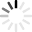# Square Quizzes & Trivia

The square is a shape of equality. A shape we are all familiar with. Do you think you know pretty much all there is to know about squares? Do you think you are ready for our square quiz? Are you ready to be dazzled by how little you don’t know about them? We’ve challenged you now, haven’t we?!

What else can a square be defined as? What is the relation between wombats and squares? Why do we call multiplying two numbers together squaring? These are just a sample of the questions you will be faced with in our original square quiz. Do you think you know the answers? Take them all now and find out! Be there or be square!
Top Trending

Practise your squares and cubes up to 20

Questions: 38  |  Attempts: 3237   |  Last updated: Jun 2, 2016

Do rhombus and squares confuse you? Having covered their properties in class today the answer to that question should be No. If the answer is yes give the quiz below a try and see what you did not understand today and ask for...

Questions: 10  |  Attempts: 163   |  Last updated: Jun 25, 2018
• Sample Question
Which of the following are true about a rhombus?I. The diagonals are perpendicular.II. The sides are all congruent.III. The diagonals are congruent.A rhombus and a square are both quadrilaterals in which all sides are equal. However, the mathematical equations for these two shapes are different. Take this first version questions on the properties of the rhombi and...

Questions: 10  |  Attempts: 311   |  Last updated: Jun 25, 2018
• Sample Question
Which of the following are true about a rhombus?This quiz covers all areas of completing the square

Questions: 5  |  Attempts: 778   |  Last updated: Jan 11, 2013
• Sample Question
What is the first thing that should be done to complete the square on 4x^2+8x+16=0Do you feel uneasy when it comes to simplifying square roots? Not any more. Play this quiz and simplify square roots easily.

Questions: 8  |  Attempts: 215   |  Last updated: Dec 9, 2016
• Sample Question
What is the basic thing to follow in order to simplify a square root?Related